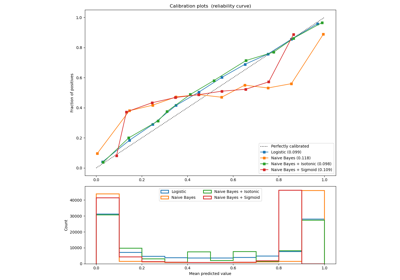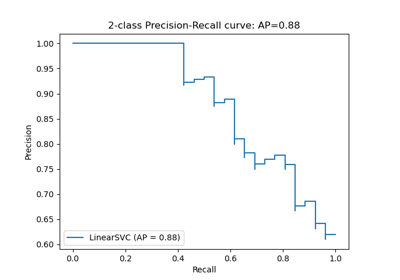# sklearn.metrics.recall_score¶

sklearn.metrics.recall_score(y_true, y_pred, *, labels=None, pos_label=1, average='binary', sample_weight=None, zero_division='warn')[source]

Compute the recall

The recall is the ratio tp / (tp + fn) where tp is the number of true positives and fn the number of false negatives. The recall is intuitively the ability of the classifier to find all the positive samples.

The best value is 1 and the worst value is 0.

Read more in the User Guide.

Parameters
y_true1d array-like, or label indicator array / sparse matrix

Ground truth (correct) target values.

y_pred1d array-like, or label indicator array / sparse matrix

Estimated targets as returned by a classifier.

labelslist, optional

The set of labels to include when average != 'binary', and their order if average is None. Labels present in the data can be excluded, for example to calculate a multiclass average ignoring a majority negative class, while labels not present in the data will result in 0 components in a macro average. For multilabel targets, labels are column indices. By default, all labels in y_true and y_pred are used in sorted order.

Changed in version 0.17: parameter labels improved for multiclass problem.

pos_labelstr or int, 1 by default

The class to report if average='binary' and the data is binary. If the data are multiclass or multilabel, this will be ignored; setting labels=[pos_label] and average != 'binary' will report scores for that label only.

averagestring, [None, ‘binary’ (default), ‘micro’, ‘macro’, ‘samples’, ‘weighted’]

This parameter is required for multiclass/multilabel targets. If None, the scores for each class are returned. Otherwise, this determines the type of averaging performed on the data:

'binary':

Only report results for the class specified by pos_label. This is applicable only if targets (y_{true,pred}) are binary.

'micro':

Calculate metrics globally by counting the total true positives, false negatives and false positives.

'macro':

Calculate metrics for each label, and find their unweighted mean. This does not take label imbalance into account.

'weighted':

Calculate metrics for each label, and find their average weighted by support (the number of true instances for each label). This alters ‘macro’ to account for label imbalance; it can result in an F-score that is not between precision and recall.

'samples':

Calculate metrics for each instance, and find their average (only meaningful for multilabel classification where this differs from accuracy_score).

sample_weightarray-like of shape (n_samples,), default=None

Sample weights.

zero_division“warn”, 0 or 1, default=”warn”

Sets the value to return when there is a zero division. If set to “warn”, this acts as 0, but warnings are also raised.

Returns
recallfloat (if average is not None) or array of float, shape = [n_unique_labels]

Recall of the positive class in binary classification or weighted average of the recall of each class for the multiclass task.

Notes

When true positive + false negative == 0, recall returns 0 and raises UndefinedMetricWarning. This behavior can be modified with zero_division.

Examples

>>> from sklearn.metrics import recall_score
>>> y_true = [0, 1, 2, 0, 1, 2]
>>> y_pred = [0, 2, 1, 0, 0, 1]
>>> recall_score(y_true, y_pred, average='macro')
0.33...
>>> recall_score(y_true, y_pred, average='micro')
0.33...
>>> recall_score(y_true, y_pred, average='weighted')
0.33...
>>> recall_score(y_true, y_pred, average=None)
array([1., 0., 0.])
>>> y_true = [0, 0, 0, 0, 0, 0]
>>> recall_score(y_true, y_pred, average=None)
array([0.5, 0. , 0. ])
>>> recall_score(y_true, y_pred, average=None, zero_division=1)
array([0.5, 1. , 1. ])


## Examples using sklearn.metrics.recall_score¶